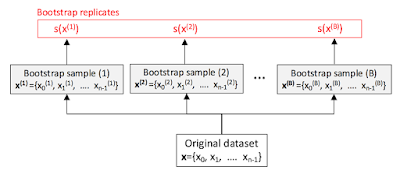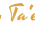## Thursday, May 5, 2016

### Bootstrapping by resampling with replacement

Target audience: Intermediate
Estimated reading time: 20'

Bootstrapping is a statistical resampling method that consists of randomly sampling a dataset with replacement. This technique enables data scientists to estimate the sampling distribution of a wide variety of probability distributions.

Background
One key objective of bootstrapping is to compute the accuracy of any statistic such as mean, standard deviation, median, mode or error. These statistics, s are known as estimators of an approximate distribution. The most common approximate distribution is known as the empirical distribution function. In the case, the observations are independent and evenly distributed (iid), the empirical or approximate distribution can be estimated through resampling.
The following diagram captures the essence of bootstrapping by resampling.Generation of bootstrap replicates by resampling

Each of the B bootstrap samples has the same number of observations or data points as the original dataset from which the samples are created. Once the samples are created, a statistical function s such as mean, mode, median or standard deviation is computed for each sample.
The standard deviation for the B statistics should be similar to the standard deviation of the original dataset.

Implementation in Scala
The purpose of this post is to illustrate some basic properties of bootstrapped sampling
• Profile of the distribution of statistics s for a given probability distribution
• Comparison of the standard deviation of the statistics s with the standard deviation of the original dataset
Let's implement a bootstrap by resampling in Scala, starting with a class Bootstrap.

  1 2 3 4 5 6 7 8 9 10 11 12 13 14 15 16 17 18 class Bootstrap( numSamples: Int = 1000, s: Vector[Double] => Double, inputDistribution: Vector[Double], randomizer: Random ) { lazy val bootstrappedReplicates: Array[Double] = ( 0 until numSamples )./:( new mutable.ArrayBuffer[Double] )( ( buf, _ ) => buf += createBootstrapSample ).toArray def createBootstrapSample: Double {} lazy val mean = bootstrappedReplicates.reduce( _ + _ )/numSamples def error: Double = {} } 

The class Bootstrap is instantiated with a predefine number of samples, numSamples (line 2), a statistic function s (line 3), a dataset generated by a given distribution inputDistribution (line 4) and a randomizer (line 5).
The computation of the bootstrap replicates, bootstrappedReplicates is central to resampling (lines 8 - 11). As described in the introduction, a replicate, s is computed from a sample of the original data set with the method createBootstrapSample (line 10).

Let's implement the method createBootstrapSample.

  1 2 3 4 5 6 7 8 9 10 def createBootstrapSample: Double = s( ( 0 until inputDistribution.size )./:( new mutable.ArrayBuffer[Double] )( ( buf, _ ) => { randomizer.setSeed( randomizer.nextLong ) val randomValueIndex = randomizer.nextInt( inputDistribution.size ) buf += inputDistribution( randomValueIndex ) } ).toVector ) 

The method createBootstrapSample
- Samples the original dataset using a uniform random function (line 6)
- Applies the statistic function s to this sample dataset (line 1 & 11)

The last step consists of computing the error (deviation) on the bootstrap replicates

 1 2 3 4 5 6 7 8  def error: Double = { val sumOfSquaredDiff = bootstrappedReplicates.reduce( (s1: Double, s2: Double) => (s1 - mean) (s1 - mean) + (s2 - mean)*(s2 - mean) ) Math.sqrt(sumOfSquaredDiff / (numSamples - 1)) } 

Evaluation
The first evaluation consists of comparing the distribution of replicates with the original distribution. To this purpose, we generate an input dataset using
• Normal distribution
• LogNormal distribution
Let's create a method, bootstrapEvaluation to compare the distribution of the bootstrap replicates with the dataset from which the bootstrap samples are generated.

  1 2 3 4 5 6 7 8 9 10 11 12 13 14 15 16 17 18 19 20 21 22 23 24 25 26 27 28 def bootstrapEvaluation( dist: RealDistribution, random: Random, gen: (Double, Double) ): (Double, Double) = { val inputDistribution = (0 until 5000)./:(new ArrayBuffer[(Double, Double)]) ( ( buf, _ ) => { val x = gen._1 * random.nextDouble - gen._2 buf += ( ( x, dist.density( x ) ) ) } ).toVector val mean = (x: Vector[Double]) => x.sum/x.length val bootstrap = new Bootstrap( numReplicates, mean, inputDistribution.map( _._2 ), new Random( System.currentTimeMillis) ) val meanS = bootstrap.bootstrappedReplicates.sum / bootstrap.bootstrappedReplicates.size val sProb = bootstrap.bootstrappedReplicates.map(_ - meanS) // .. plotting histogram of distribution sProb (bootstrap.mean, bootstrap.error) } 

We are using the normal and log normal probability density function defined in the Apache Commons Math Java library. These probability density functions are defined in the org.apache.commons.math3.distribution package.
The comparative method bootstrapEvaluation has the following argument:

• dist: A probability density function used to generate the dataset upon which sampling is performed (line 2).
• random: A random number generator (line 3)
• gen: A pair of parameters for the linear transform for the generation of random values (a.r + b) (line 4).
The input distribution inputDistribution { (x, pdf(x)} is generated for 5000 data points (lines 7 - 13).
Next the bootstrap is created with the appropriate number of replicates, numReplicates, the mean of the input dataset as the statistical function s, the input distribution and the generic random number generator of Scala library, as arguments (lines 16 -20).
Let's plot the distribution the input dataset generated from a normal density function.

val (meanNormal, errorNormal) = bootstrap(
new NormalDistribution,
new scala.util.Random,
(5.0, 2.5)
)


Normally distributed dataset
The first graph plots the distribution of the input dataset using the Normal distribution.

The second graph illustrates the distribution (histogram) of the replicates s - mean.

The bootstrap replicates s(x) are also normally distributed. The mean value for the bootstrap replicates is 0.1978 and the error is 0.001691

Dataset with a log normal distribution
We repeat the same process for the lognormal distribution. This time around the dataset to sample from follows a log-normal distribution.

val (meanLogNormal, errorLogNormal) = bootstrap(
new LogNormalDistribution,
new scala.util.Random,
(2.0, 0.0)
)


Although the original dataset used for generated the bootstrap samples is normally distribured, the bootstrap replicates s(x) are normally distributed. The mean for the bootstrap replicates is 0.3801 and the error is 0.002937

The error for the bootstrap resampling from a log normal distribution is twice as much as the error related to the normal distribution
The results is not be a surprise: The bootstrap replicates follow a Normal distribution which matches closely the original dataset created using the same probability density function.

References
• Programming in Scala - 3rd edition M Odersky, L. Spoon, B. Venners - Artima - 2016
• Elements of Statistics Learning: Data mining, Inference and Prediction - 7.11 Bootstrap method Springer - 2001

1.Very interesting resampling implementation in Scala. Something that will keep the students in term paper help pre-occupied with this challenge.

2.Thanks for sharing helpful information, I really like your all post. I will bookmark your blog for future updates. Send Gift To Pakistan

3.4.This comment has been removed by the author.

5.6.7.مكافحة حشرات بالخبر مكافحة حشرات بالخبر
مكافحة حشرات بمكة مكافحة حشرات بمكة
مكافحة حشرات بالمدينة المنورة شركة مكافحة حشرات بالمدينة المنورة
مكافحة حشرات بالدمام شركة مكافحة حشرات بالدمام

8.9.10.11.Paint: Different types and colors of paints are also used for the art work. Some of the types of paints used for craft works are gloss, oil paint, spray, acrylic paint and enamel. Glitter for crafts

12.Hi, I'm Alex Starc. I genuinely gained some remarkable experiences in assessing your article.
Send gifts to Pakistan